Riley is reading a book that has twenty pages. Riley reads twelve pages before lunch. Riley reads five pages after lunch. Riley says she read seventeen pages in her book. Is Riley correct? Show all your mathematical thinking.

Assessment

Plan

Formal Mathematical Language and Symbolic Notation

A student may independently select a printed number line, number chart, ten frames, graph paper, etc. as they work on a task.

Suggested materials

Engagement Image:

Teachers may project the image below to launch this task for their students, define nouns, promote discussion, access prior knowledge, and inspire engagement and problem solving.

Solutions tabs

Expert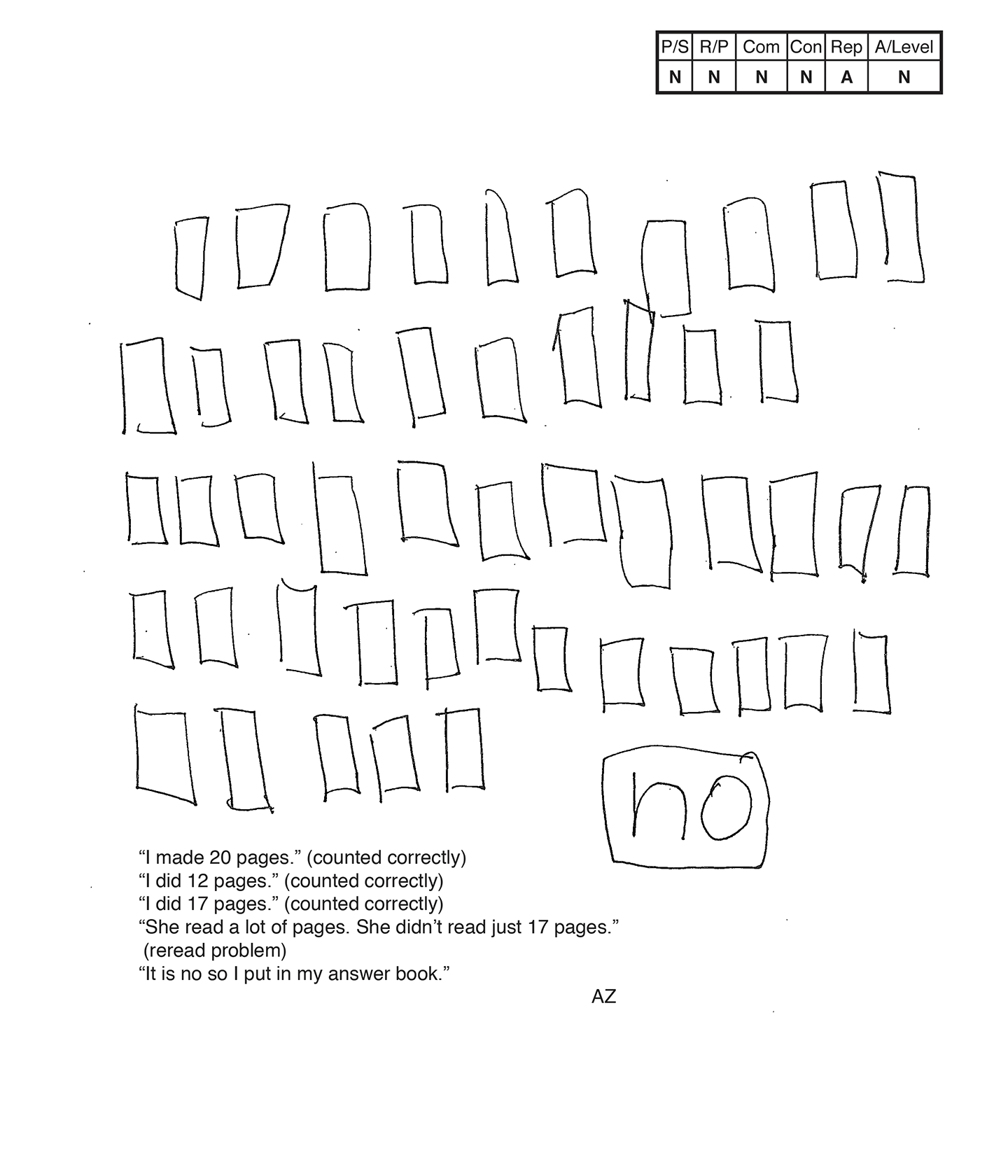This student does not meet the standard.

Scoring Rationale

Novice

The student's strategy of diagramming 49 pages would not work to solve the task. The student's answer, "no," is not correct.

Novice

The student does not show correct reasoning of the underlying concept of addition. The student does not combine a set of 12 pages with a set of five pages to determine if the total pages read was 17.

Novice

The student does not use any mathematical language to communicate their reasoning and proof.

Novice

The student does not make a mathematically relevant observation about their solution.

Apprentice

The student attempts to make a diagram, but it is not accurate. The scribing states that the student first diagrams 20 pages, 12 pages, and 17 pages.

Novice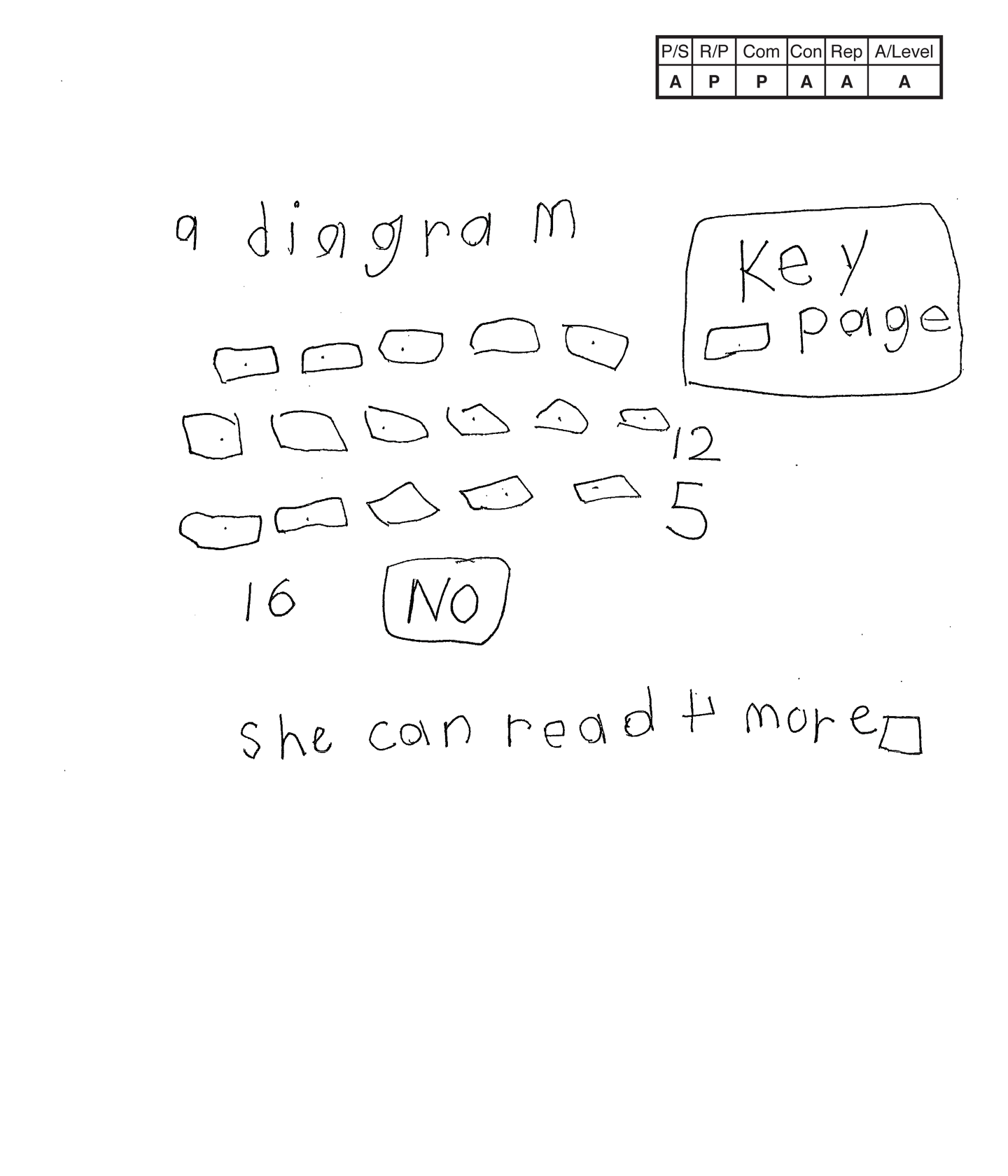This student does not meet the standard.

Scoring Rationale

Apprentice

The student's strategy of diagramming 12 and five pages would work to solve the task. The student's answer, "No," is not correct.

Practitioner

The student shows correct reasoning of the underlying concept of addition. The student understands that the task requires adding 12 and five pages to determine if the total is 17. The student diagrams only 11 pages in the first two rows, which causes an addition error that leads to an incorrect answer.

Practitioner

The student correctly uses the mathematical terms diagram and key. The student does not earn credit for the term more, as the student's connection is incorrect.

Apprentice

The student attempts to make a mathematically relevant observation about their solution, "She can read 4 more (student drew a square to represent a book page)." This is an incorrect connection. The student can read three more pages.

Apprentice

The student's strategy of diagramming pages read is appropriate to the task but is not accurate. The student only diagrams 11 pages instead of 12 in the first two rows. The student provides a key to define the pages.

Apprentice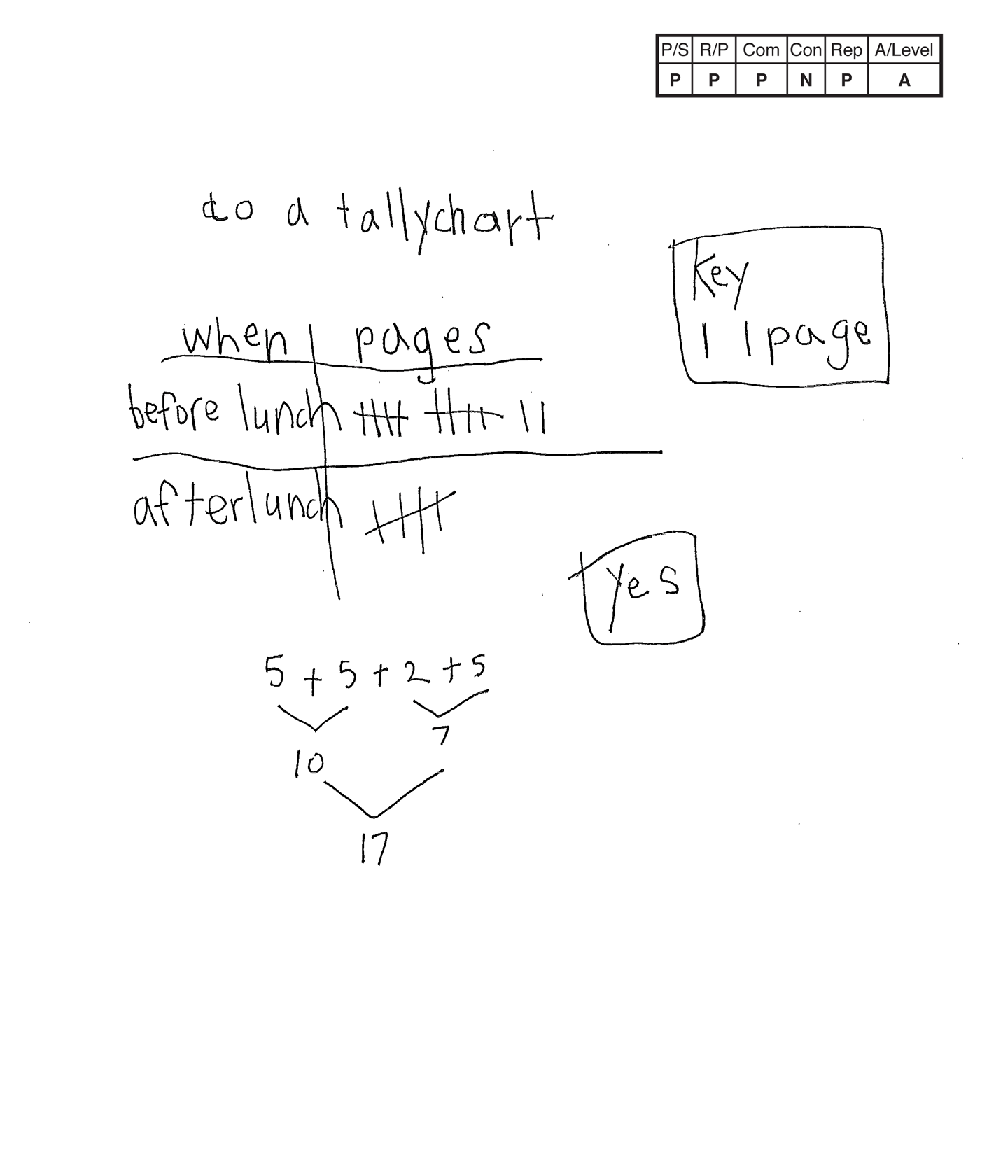This student does not meet the standard.

Scoring Rationale

Practitioner

The student's strategy of using a tally chart to indicate the pages read before and after lunch works to solve the task. The student's answer, "yes," is correct.

Practitioner

The student shows correct reasoning of the underlying concept of addition. The student understands that the task requires adding 12 and five pages to determine if the total is 17.

Practitioner

The student correctly uses the mathematical terms tally chart, key, before, after.

Novice

The student solves the problem and stops without making a mathematically relevant observation.

Practitioner

The student's tally chart is appropriate to the task and accurate. A key defines each tally mark as a page and all entered data is accurate.

Apprentice

Note:

The overall achievement level for this piece of student work falls under Exemplars exception to the rule category. If a student has all Apprentice scores or above, but a Novice in "Connections," the student may still receive an achievement level score of Apprentice. To learn more about Exemplars scoring, please refer to the section of your dashboard called "Tools for Success" and click on the link for “Using the Assessment Rubric.”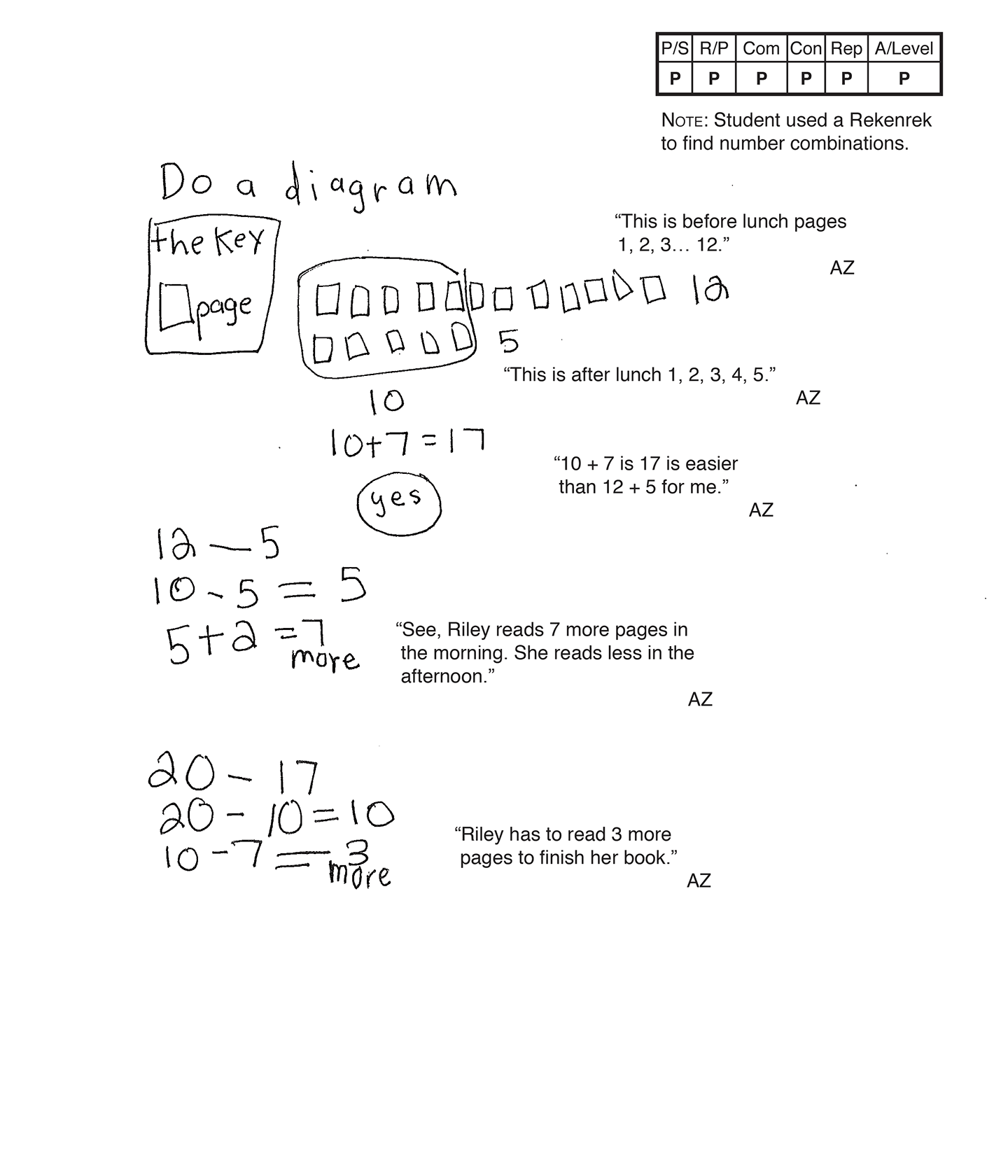This student meets the standard.

Scoring Rationale

Practitioner

The student's strategy of using a tally chart to indicate the pages read before and after lunch works to solve the task. The student's answer, "yes," is correct.

Practitioner

The student shows correct reasoning of the underlying concept of addition. The student understands that the task requires adding 12 and five pages to determine if the total is 17.

Practitioner

The student correctly uses the mathematical terms diagram, key, before, after, more, less.

Practitioner

The student makes mathematically relevant observations. The student writes 12 – 5, 10 – 5 = 5, 5 + 2 = 7 more. The student states, "See, Riley reads seven more pages in the morning. She reads less in the afternoon." The student writes 20 – 17, 20 – 10 = 10, 10 – 7 = 3 more. The student states, "Riley has to read three more pages to finish her book."

Practitioner

The student's diagram is appropriate to the task and accurate. A key defines the pages. The scribing also indicates that the student uses a Rekenrek to model their number combinations in their connections.

Practitioner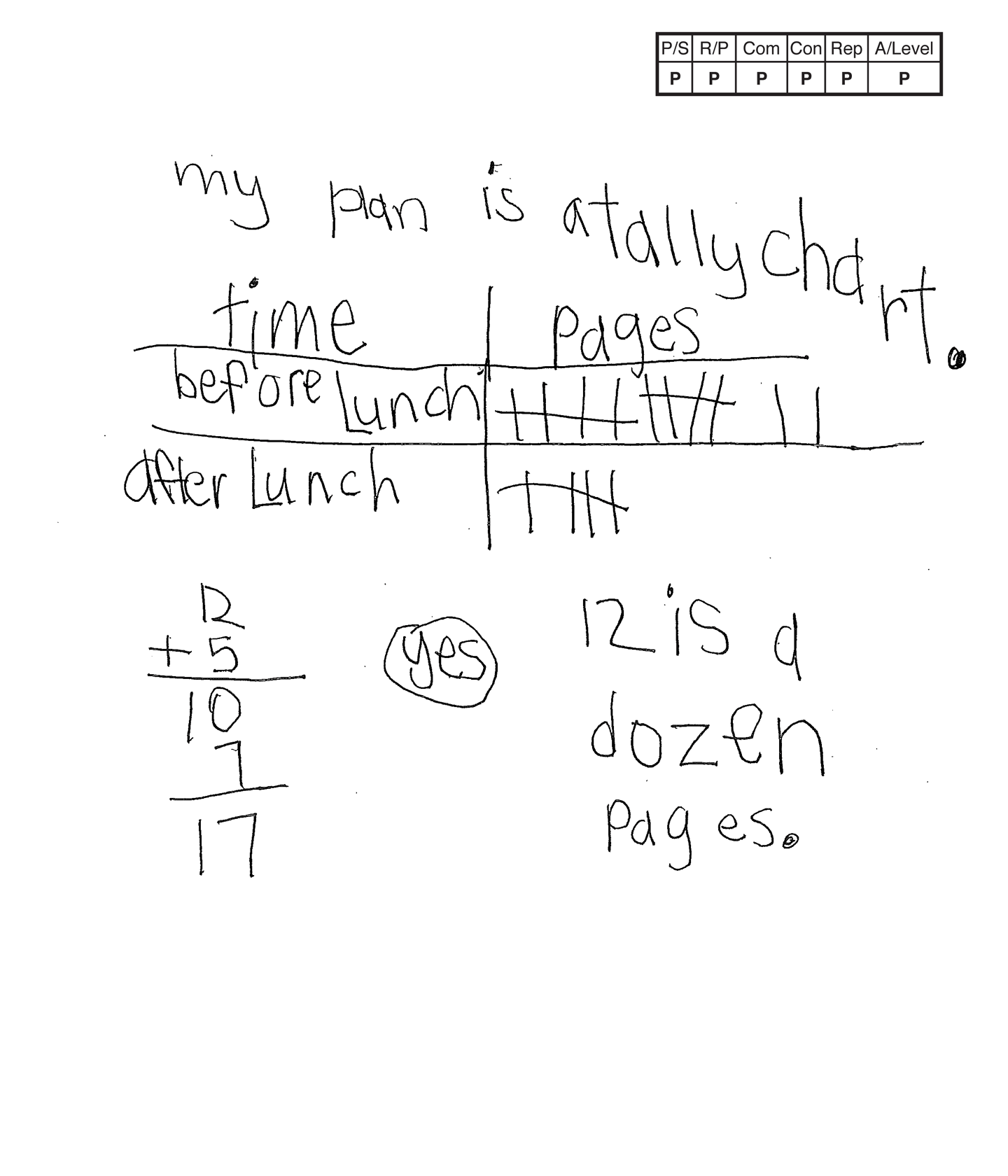This student meets the standard.

Scoring Rationale

Practitioner

The student's strategy of using a tally chart to indicate the pages read before and after lunch works to solve the task. The student's answer, "yes," is correct.

Practitioner

The student shows correct reasoning of the underlying concept of addition. The student understands that the task requires adding 12 and five pages to determine if the total is 17.

Practitioner

The student correctly uses the mathematical terms tally chart, time, before, after, dozen.

Practitioner

The student makes a mathematically relevant observation, "12 is a dozen pages."

Practitioner

The student's tally chart is appropriate to the task and accurate. If a tally mark represents one (page), a key is not necessary.

Practitioner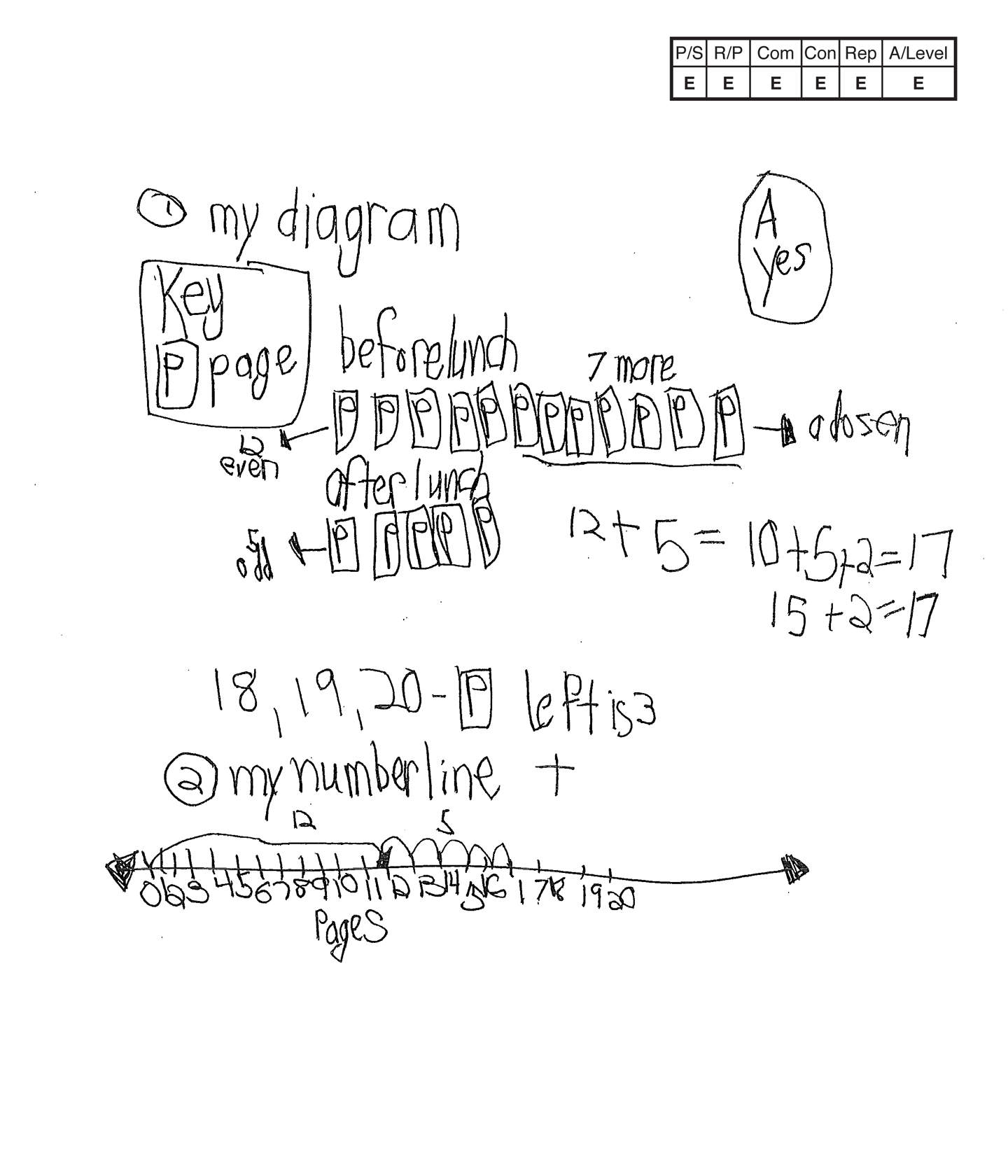,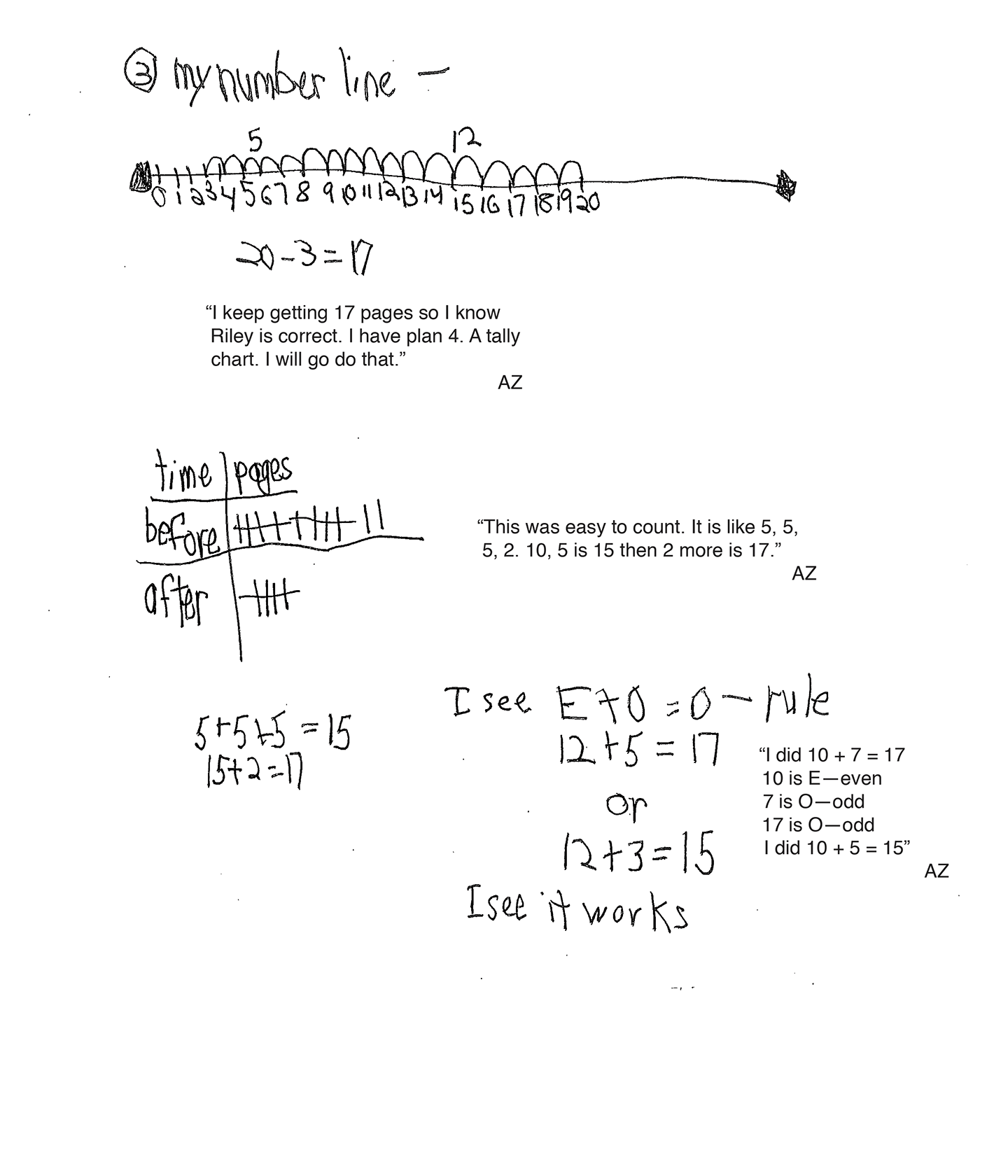This student exceeds the standard.

Scoring Rationale

Expert

The student's strategy of using a diagram to indicate the pages read before and after lunch works to solve the task. The student's answer, "Yes," is correct. The student verifies their answer with two number lines and a tally chart. The student also explores the Even + Odd = Odd rule.

Expert

The student shows correct reasoning of the underlying concept of addition. The student understands that the task requires adding 12 and five pages to determine if the total is 17. The student verifies their answer and explains the Even + Odd = Odd rule.

Expert

The student correctly uses the mathematical terms diagram, key, before, after, more, dozen, even, odd, number line, tally chart, time, rule.

Expert

The student makes the mathematically relevant observations, "a dozen," "12 even," "5 odd," and, "18, 19, 20-p left is 3." The student verifies their answer by using a number line to show 12 pages plus five pages, and a number line to show 20 pages subtract three pages with the number sentence 20 – 3 = 17. The student states, "I keep getting 17 pages so I know Riley is correct." The student then states, "I have plan 4. A tally chart. I will go do that." The student completes a tally chart and states, "This was easy to count. It is like 5, 5, 5, 2. 10, 5 is 15 then 2 more is 17." The student then states a rule, "E + O = O." The student shows how this rule is correct with 12 + 5 = 17 and 12 + 3 = 15 and states, "I see it works."

Expert

The student's diagram is appropriate to the task and accurate. A key defines the pages. The student's two number lines are also appropriate to the problem and accurate. The numbers are defined as pages and the student correctly "jumps" each page correctly. The student also creates an appropriate and accurate tally chart. The student uses the number lines and tally chart to verify that the student's answer is correct.